# Interesting Probability Examples Set 2

Go back to  'Probability'

Example – 25

A large chart consists of parallel lines drawn at unit spacings. You drop a needle of length 1 somewhere on this chart. What is the probability that the needle will not cross any line?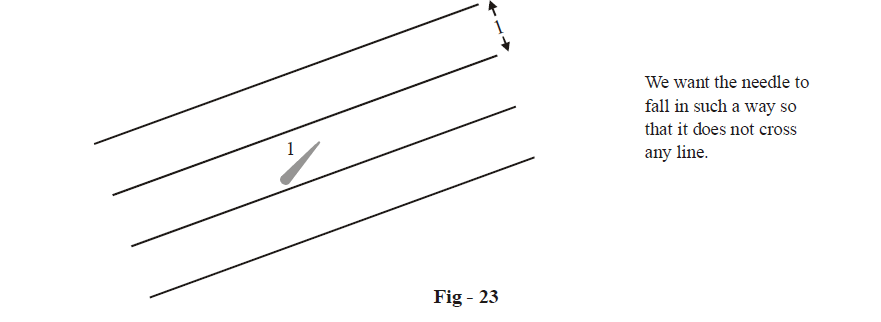Solution: Once again, this is a problem of continuous distribution: there are two variables with respect to the needle-the distance x of the centre of the needle from the closest line, and the angle

$$\theta$$ relative to the parallel lines at which the needle rests after falling.

x can vary uniformly in  \begin{align}\left[ 0,\ \frac{1}{2} \right]\end{align}, because xmin = 0 occurs when the centre of the needle falls on a line, while \begin{align}{{x}_{\max }}=\frac{1}{2}\end{align} occurs when the centre of the needle falls exactly midway between two lines.

$$\theta$$ can vary uniformly in $$\left[ 0,\ \pi \right)$$ , which should be obvious.

Thus, the ‘total’ region or the sample space for the experiment is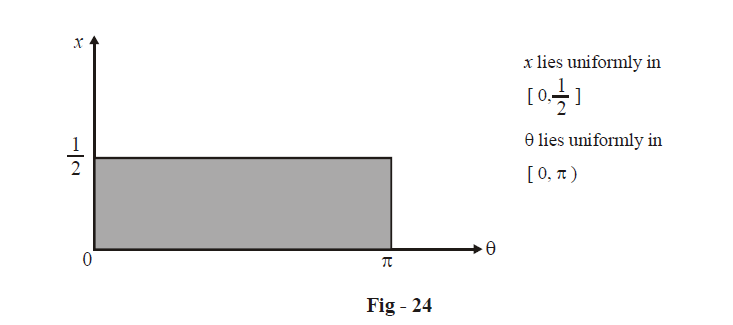To determine the ‘favorable’ region, that is, those ($$\theta$$, x) for which the needle does not cross any line, consider the following figure: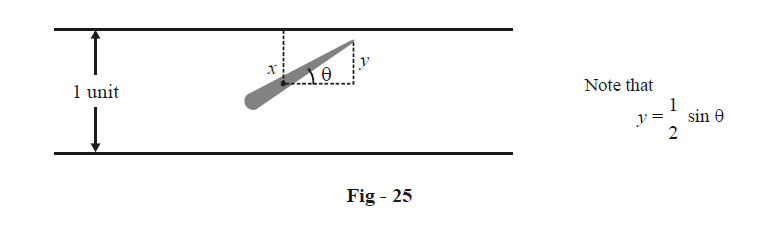The needle will miss the lines only if

$x\ge y$

i.e,

\left\{ \begin{gathered} \text{The distance of the } \\ \text{centre of the needle} \\ \text{from the closest line} \\ \end{gathered} \right\}\ \ \ge \ \ \left\{ \begin{align} & \text{The perpendicular} \\ & \text{component of half} \\ & \text{the needle} \\ \end{align} \right\}

For example, in the following scenario,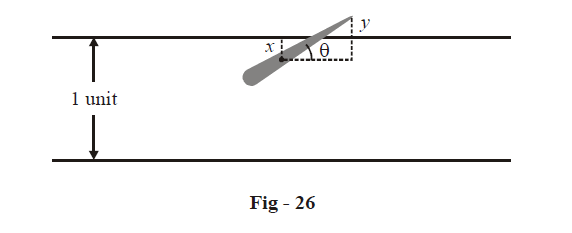the needle crosses a line because x < y.

Thus, the favorable condition is  $$x \ge y$$ or

$x \ge \frac{1}{2}\sin \theta$

Let us plot this region within the total sample space in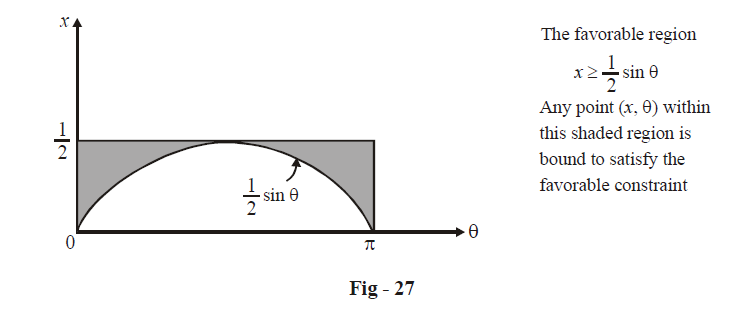The area of this shaded region is  \begin{align}\frac{1}{2}\pi - \int\limits_0^\pi {\frac{1}{2}} \sin \theta \;d\;\theta =\frac{1}{2}\pi -1\end{align}

Finally, the required probability p is

\begin{align}&p = \frac{{{\text {Area of favorable region}}}}{{{\text{Area of total region}}}}\\\\&\; = \frac{{\frac{1}{2}\pi - 1}}{{\frac{1}{2}\pi }}\\\\&\;= 1 - \frac{2}{\pi }\\\\&\;= 0.363\end{align}

Thus, there is a 36.3% chance that the needle will not cross any line

There is an interesting story behind this problem: it was first posed in the 18th century by a mathematician called Buffon, and is hence known as the Buffon’s needle problem. The general problem involves parallel lines at t units of spacing and a needle of length l. You are urged to show that the general result is \begin{align}1 - \frac{{2l}}{{t\pi }}\end{align} which reduces to  \begin{align}1 - \frac{2}{\pi }\end{align} in the case we discussed, i.e., t = l

Note that the expression for the probability contains $$\pi$$ . In particular, we have

\begin{align} & \frac{2l}{t\pi }=P(\text{needle}\ \text{crosses}\ \text{a}\ \text{line}) \\ &\quad\; \approx \frac{\text{Number of times needle crosses a line in }n\text{ throws}}{n} \\ &\qquad\quad \left( \text{where n is large} \right) \\ \end{align}

If we call a needle crossing a line as a hit, we have

$\pi \approx \frac{2ln}{t\cdot (\text{No}\text{.}\ \text{of}\ \text{hits})}$

This can be used as a crude way of approximating the value of $$\pi$$ . In fact, in 1901, an Italian mathematician called Lazzarini tossed a needle 3408 times and obtained an estimate of $$\pi$$  as \begin{align}\frac{355}{113}\end{align} . More information about this subject is available online.

Example – 26

We now come to publicly one of the most well known problems in probability. This version of the problem and its solution have been taken from Wikipedia.

Suppose you are in a game show, and you’re given the choice of three doors. Behind one door is a new car; behind the others, goats.The car and the goats were placed randomly behind the doors before the show. The rules of the game show are as follows: After you’ve chosen a door, the door remains closed for the time being. The game show host, Monty Hall, who knows what is behind the doors, now has to open one of the two remaining doors, and the door he opens must have a goat behind it. If both remaining doors have goats behind them, he chooses one randomly. After Monty Hall opens a door with a goat, he will ask you to decide whether you want to stay with your first choice or to switch to the last remaining door. Imagine that you choose Door 1 and the host opens Door 3, which has a goat. He then asks you “Do you want to switch to Door 2?” Is it to your advantage to change your choice?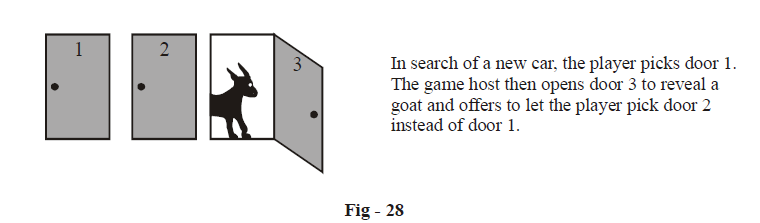Solution: Before going through the solution, give the problem a great deal of thought. Will switching change the probability of wining? Or will it not matter because it is equally likely that the car may be behind Door 1 or Door 2 ?

O.K., now the solution. We are assuming that the player initially picked Door 1. (However, the player may initially choose any of the three doors). We’ll now calculate the probability of winning by switching, by listing out all cases explicitly.

Case 1: The car is behind Door 1. In this case, the game host Monty Hall must open one of the two remaining doors randomly

Case 2: The car is behind Door 2. Then the host must open Door 3

Case 3: The car is behind Door 3. Then the host must open Door 2

If the player chooses to switch, he’ll win only if the car is behind either of the two unchosen doors rather than the one that was originally picked.

The following diagram visually depicts the various cases and what happens with switching.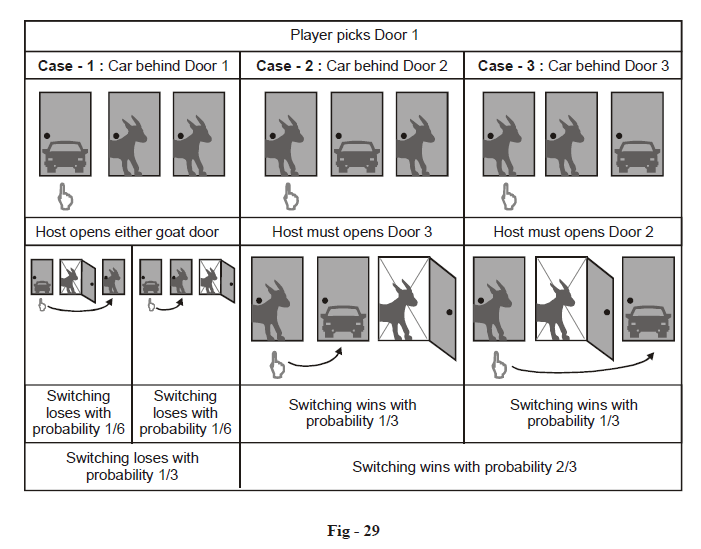Let us explain how we arrived at the various probabilities using a probability tree: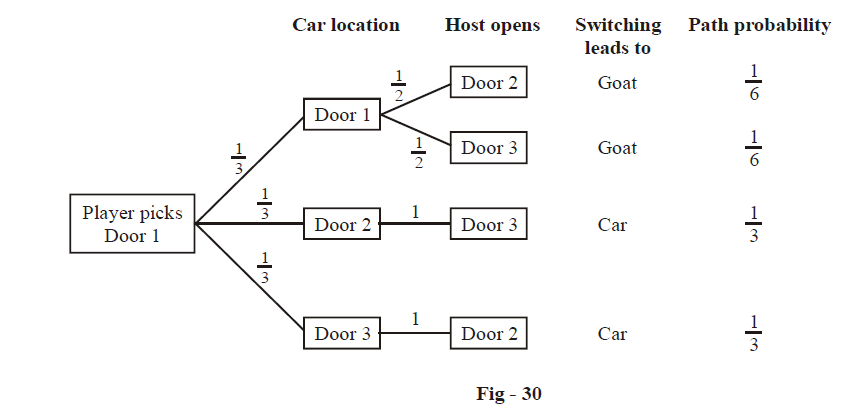Thus, switching leads to winning with probability \begin{align}\frac{2}{3}\end{align} , that is, the player should switch for a higher probability of winning!

This result may seem very counter-intuitive to you. After all, you may say, the remaining two doors must each have a probability  \begin{align}\frac{1}{2}\end{align}  of containing the car. However, this intuition is wrong.

In fact, you are not alone in this intuition . When first presented with this problem, an overwhelming majority of people assume that each door has an equal probability and conclude that switching does not matter. Even Nobel laureates have been known to have given the wrong answer and to have insisted upon it! For more interesting facts about this problem, you’ll find a lot of resources online.

Open-ended question (for discussion)

Q. 1 Here is a problem very similar to the Monty Hall Problem.

There are three condemned prisoners, a random one of whom has been secretly chosen to be pardoned. One of the prisoner begs the warden to tell him of one of the others who will be executed, arguing that this reveals no information about his own fate, but increases his chances of being pardoned from \begin{align}\frac{1}{3}\end{align} to \begin{align}\frac{1}{2}\end{align} . The warden obliges, secretly flipping a coin to decide which name to provide if the prisoner who is asking is the one being pardoned. Does the warden’s answer changes the prisoner’s chances of being pardoned?

Q. 2     (a) Is it ‘possible’ that you may obtain a sequence of a 100 Heads in successively tossing a fair coin? How long will you have to wait before there is a reasonable chance that such a thing might occur.

(b) A computer produces a random letter each picosecond. Can you estimate the probability of this sequence of random letters containing the entire text of Shakespeare’s Hamlet?

Q. 3 If you are made the head of an international think-tank, how will you estimate the probability of a world war breaking out the next month?